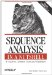# lindna

 lindna

lindna draws linear maps of DNA constructs.

Here is a sample session with lindna:

`% lindna Draws linear maps of DNA constructs Graph type [x11]: Input file [inputfile]: data.linp do you want a ruler (Y or N) [Y]: type of blocks (enter Open, Filled, or Outline) [Filled]:`

#### Mandatory qualifiers:

-graphout (graph)

Graph type.

-inputfile (infile)

Input file containing mapping data.

-ruler (string)

Do you want a ruler (Y or N)?

-blocktype (string)

Type of blocks: Open, Filled, or Outline. Option Outline draws filled blocks surrounded by a black border.

#### Optional qualifiers:

-intersymbol (string)

Type of junctions between blocks (one of Straight, Up, Down, or No).

-intercolor (integer)

Color of junctions between blocks (enter a color number).

-interticks (string)

Do you want horizontal junctions between ticks (Y or N)?

-gapsize (integer)

Interval between ticks in the ruler (enter an integer).

-ticklines (string)

Do you want vertical lines at the ruler's ticks (Y or N)?

-textheight (float)

Height of text. Enter a number less than 1 to decrease the size. Enter a number greater than 1 to increase the size.

-textlength (float)

Length of text. Enter a number less than 1 to decrease the size. Enter a number greater than 1 to increase the size.

-margin (float)

Width of left margin. This is the region left of the groups where the names of the groups are displayed. Enter a number less than 1 to decrease the size. Enter a number greater than 1 to increase the size.

-tickheight (float)

Height of ticks. Enter a number less than 1 to decrease the size. Enter a number greater than 1 to increase the size.

-blockheight (float)

Height of blocks. Enter a number less than 1 to decrease the size. Enter a number greater than 1 to increase the size.

-rangeheight (float)

Height of range ends. Enter a number less than 1 to decrease the size. Enter a number greater than 1 to increase the size.

-gapgroup (float)

Space between groups. Enter a number less than 1 to decrease the size. Enter a number greater than 1 to increase the size.

-postext (float)

Space between text and ticks, blocks, and ranges. Enter a number less than 1 to decrease the size. Enter a number greater than 1 to increase the size.Sequence Analysis in a Nutshell: A Guide to Common Tools and Databases
ISBN: 059600494X
EAN: 2147483647
Year: 2005
Pages: 312

Similar book on Amazon